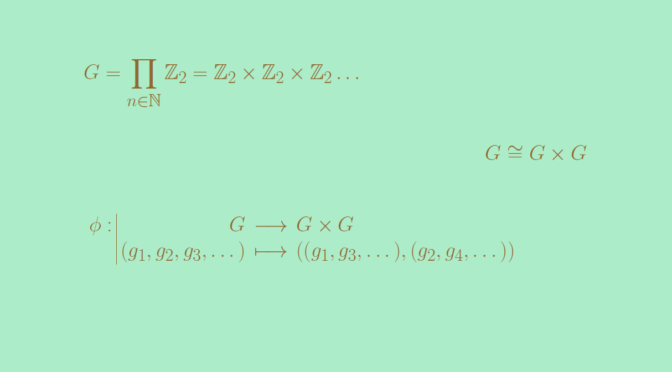A group G isomorph to the product group G x G

Let’s provide an example of a nontrivial group $$G$$ such that $$G \cong G \times G$$. For a finite group $$G$$ of order $$\vert G \vert =n > 1$$, the order of $$G \times G$$ is equal to $$n^2$$. Hence we have to look at infinite groups in order to get the example we’re seeking for.

We take for $$G$$ the infinite direct product $G = \prod_{n \in \mathbb N} \mathbb Z_2 = \mathbb Z_2 \times \mathbb Z_2 \times \mathbb Z_2 \dots,$ where $$\mathbb Z_2$$ is endowed with the addition. Now let’s consider the map $\begin{array}{l|rcl} \phi : & G & \longrightarrow & G \times G \\ & (g_1,g_2,g_3, \dots) & \longmapsto & ((g_1,g_3, \dots ),(g_2, g_4, \dots)) \end{array}$

From the definition of the addition in $$G$$ it follows that $$\phi$$ is a group homomorphism. $$\phi$$ is onto as for any element $$\overline{g}=((g_1, g_2, g_3, \dots),(g_1^\prime, g_2^\prime, g_3^\prime, \dots))$$ in $$G \times G$$, $$g = (g_1, g_1^\prime, g_2, g_2^\prime, \dots)$$ is an inverse image of $$\overline{g}$$ under $$\phi$$. Also the identity element $$e=(\overline{0},\overline{0}, \dots)$$ of $$G$$ is the only element of the kernel of $$G$$. Hence $$\phi$$ is also one-to-one. Finally $$\phi$$ is a group isomorphism between $$G$$ and $$G \times G$$.